### Create an Account

Home / Questions / Federal regulations set an upper limit of 50 parts per million (ppm) of NH3 in the air in ...

# Federal regulations set an upper limit of 50 parts per million (ppm) of NH3 in the air in a work environment

Federal regulations set an upper limit of 50 parts per million (ppm) of NH3 in the air in a work environment [that is, 50 molecules of NH3(g) for every million molecules in the air]. Air from a manufacturing operation was drawn through a solution containing 1.00 × 102 mL of 0.0105 M HCl. The NH3 reacts with HCl according to:

NH3(aq) + HCl(aqNH4Cl(aq)

After drawing air through the acid solution for 10.0 min at a rate of 10.0 L/min, the acid was titrated. The remaining acid needed 13.1 mL of 0.0588 M NaOH to reach the equivalence point. (a) How many grams of NH3 were drawn into the acid solution? (b) How many ppm of NH3 were in the air? (Air has a density of 1.20 g/L and an average molar mass of 29.0 g/ mol under the conditions of the experiment.) (c) Is this manufacturer in compliance with regulations?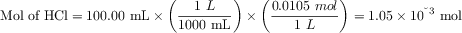After bubbling the air through the acid solution, remaining amount of acid is calculated from titration with NaOH:

HCl(aq) + NaOH(aqNaCl(aq) + H2O(l)

Mol of HCl in excess: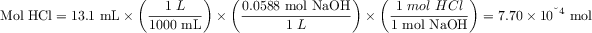The amount of HCl consumed for neutralization of ammonia from air is: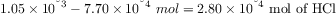The same amount of NH3 was also present in a sample, as ammonia and HCl react in 1:1 ratio.

Molar mass of NH3 is 17.0 g/mol, and the mass drawn into acid is: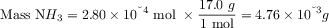b) The volume of air passed through the HCL solution is: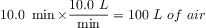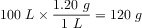Number of moles of air: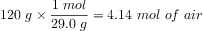The concentration of ammonia in the air, in ppm, is: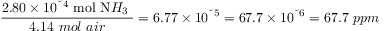c) The manufacturer is not in compliance with regulations since it releases more than 50 ppm of ammonia in the air.

Aug 23 2021 View more View LessSubscribe To Get Solution